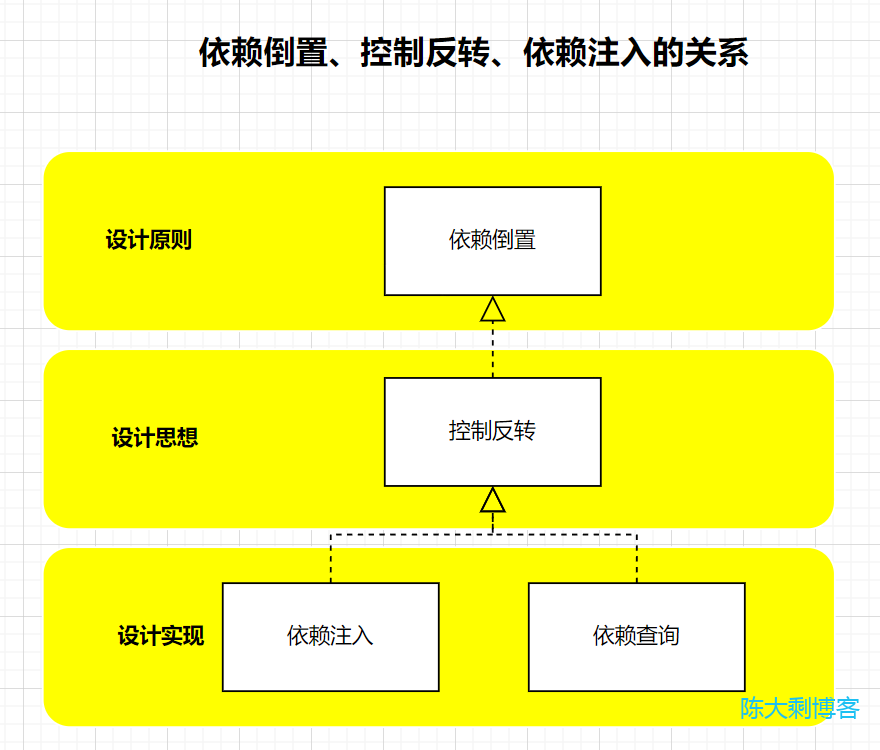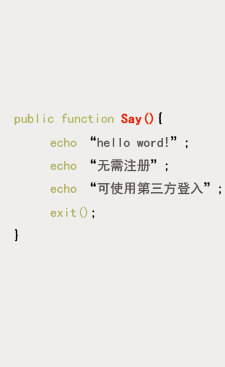# php设计模式终篇：一文读懂：依赖注入、控制反转、IoC容器

• 陈大剩
• 2023-06-16 00:07:48
• 584

## 依赖注入

### 什么是依赖？

// A 类
class A
{
public function __construct()
{
$b = new B(); // do something } } // B 类 class B { public function __construct() { return '我是 B 类'; } }  本例举例是在构造函数中依赖，除构造方法外其他方法也是一样，并不一定在构造函数中。 ### 什么是注入？ 注入 在现实生活中顾明思议是 不属于某物体的对象放入某物体中，将 A物体 注入到 B物体，如：医生给打针、汽车加油、…。在软件工程 OOP 中也是同样道理，将某个 参数/类(外部资源) 注入到另一个个类中。 注入某个参数 // A 类 class A { public function __construct($name)
{
// do something
}
}

$a = new A('cxbdasheng'); // do something  注入某个类 // A 类 class A { public function __construct($class)
{
// do something
}
}

// B 类
class B
{
public function __construct()
{
// do something
}
}

$b = new B(); // 将 B 类注入给 A 类$a = new A($b); // do something  ### 依赖注入 前面的 依赖注入 我们已经知道了，那么什么是 依赖注入 ？，依赖注入 其实是将 依赖注入 相结合。 // A 类 class A { protected$b;
public function __construct(B $class) {$this->b = $class; // do something } } // B 类 class B { public function __construct() { // do something } }$b = new B();
// 将 B 类注入给 A 类
$a = new A($b);
// do something


## 控制反转

1. 依赖倒置【设计原则】
看过设《设计模式》的同学应该对 SOLID 原则 都不陌生，其中 D(dependency Inversion Principle) 原则 也就是 依赖倒置 原则，依赖倒置原则说明：“高层次的类不应该依赖于低层次的类”。这是原则层描述。
2. 控制反转【设计思想】
控制反转 是一种 设计思想控制反转 并不指定哪种 设计实现 来实现，只要按照 控制反转 的思想去是实现，我们就称为 控制反转
3. 依赖注入【设计实现】
依赖注入，也是具体的实现，有原则和思想了总要去实现吧？也就是说 依赖注入是根据 原则(依赖倒置) 和 思想(控制反转) 来实现。根据 原则思想 去实现的还有 依赖查找，相比而言依赖注入是被动接收依赖对象，而 依赖查找主动查询依赖对象### 什么是控制？

// A 类
class A
{
public function __construct()
{
// A 控制 B
$b = new B(); // do something } } // B 类 class B { public function __construct() { return '我是 B 类'; } }  阿这……，这不就是前面依赖的例子吗？是的这个就是依赖的例子，只是在从 不同角度上描述 罢了，现在在思想层面描述，也就是 A 控制 B 。 ### 什么是反转？ 有反转那么肯定有正转，不然怎么要反转一下呢？ #### 正转 A 的实例化需要 B,直接在 A 中去实例化 B 获取 B，叫做正转。也就是说只要在 A 中实例化 B 就叫正转(不管有没有执行 B 类中的方法)。 还是上面例子 // A 类 class A { public function __construct() { // 正转$b = new B();
// do something
}
}

// B 类
class B
{
public function __construct()
{
return '我是 B 类';
}
}


#### 反转

A 类不再主动去实例化 B，而是通过一个 第三方对象(IoC 容器）,去 被动/主动 获取，等待 第三方对象 获取一个 B 的实例，叫做反转。第三方对象一般称为 IoC（Inversion of Control） 容器。

// B 类
class B
{
public function __construct()
{
return '我是 B 类';
}
}

// C 类
class C
{
public function __construct()
{
return '我是 C 类';
}
}

// IoC 容器
class IoC
{
public function getInstance($className) { switch($className)
{
case 'b':
return new B();
break;
case 'c':
return new C();
break;
default:
return null;
break;
}
}
}

// A 类
class A
{
public function __construct()
{
$ioc = new IoC();$b = $ioc->getInstance($className);
// do something
}
}


#### 为什么需要反转

A 和 B 的依赖中，A 只是想要 B 的服务。A 其实不需要管 B 的创建过程，只要有个 B 的对象来提供服务就好。

#### 通过依赖注入实现控制反转

##### 资源基类接口
/**
* 资源基类接口
*/
interface Resources
{
/**
* 使用资源
* @return mixed
* @author chendashengpc
*/
public function use(): string;
}

##### 资源具体类
/**
* A 资源
*/
class A implements Resources
{
/**
* 使用 A 资源
* @return string
* @author chendashengpc
*/
public function use(): string
{
return '现在使用的是 A 资源';
}
}

/**
* B 资源
*/
class B implements Resources
{
/**
* 使用 B 资源
* @return string
* @author chendashengpc
*/
public function use(): string
{
return '现在使用的是 B 资源';
}
}

/**
* C 资源
*/
class C implements Resources
{
/**
* 使用 C 资源
* @return string
* @author chendashengpc
*/
public function use():string
{
return '现在使用的是 C 资源';
}
}

##### IoC 容器类

IoC 容器（switch 实现）

/**
* IoC 容器（switch 实现）
*/
class Ioc
{
/**
* 获取指定资源
* @param $resources 资源名 * @return Resources * @author chendashengpc */ public function getInstance($resources): Resources
{
switch ($resources) { case 'a': return new A(); break; case 'b': return new B(); break; case 'c': return new C(); break; default: throw new \InvalidArgumentException('没有此类'); } } }  IoC 容器（反射实现） use ReflectionClass; /** * IoC 容器（反射实现） */ class ReflectorIoc { /** * 获取指定资源 * @param$resources 资源名
* @return Resources
* @author chendashengpc
*/
public function getInstance($resources): Resources { // 转化成大写类名$class = strtoupper($resources); // 反射 双引号防止转义 try {$reflector = new ReflectionClass(__NAMESPACE__ . '\\' . $class); // 调用反射类 newInstance 直接实例化了，资源类构造函数有传参需获取参数再进行实例化。$obj = $reflector->newInstance(); } catch (\Exception$exception) {
throw new \InvalidArgumentException('没有此类');
}
return $obj; } }  注：IoC 容器（反射实现）中，资源类经反射后直接实例化，并未获取资源类的参数。如资源类构造函数有传参，则需获取参数再进行实例化。 注：尽管你使用了依赖注入，但也不一定能简单替换依赖。需要在平时编码就具有高抽象思维，这也是为什么提倡依赖接口而非实现，如果平时编码是面向过程思想，就算使用了依赖注入，替换依赖仍然会是一个巨大的工程。 ##### 客户端类 /** * 使用资源的客户端 */ class Client { public Resources$resources;

public function __construct(Resources $resources) {$this->resources = $resources; } public function useResources() { echo$this->resources->use() . PHP_EOL;
}
}

$ioc = new Ioc(); /** * 将资源类注入到 Client 类中 */$client = new Client($ioc->getInstance('b'));$client->useResources();
$client = new Client($ioc->getInstance('c'));
$client->useResources();$ioc = new ReflectorIoc();
/**
* 将资源类注入到 Client 类中
*/
$client = new Client($ioc->getInstance('a'));
$client->useResources();$client = new Client($ioc->getInstance('C'));$client->useResources();


现在使用的是 B 资源



0

## 说点儿什么吧表情

## 留言墙